GeeksforGeeks App
Open AppBrowser
Continue

# How to Make Grouped Boxplot with Jittered Data Points in ggplot2 in R

In this article, we will see how to make use of ggplot2 package in R Programming Language to plot grouped boxplots with jittered data points.

Grouped Boxplots help us visualize two or more features/variables in a single plot using the grouping variable in ggplot2. The jittered points are data points that belong to another variable and are plotted on top of the grouped boxplot for a better comparison of the spread of data points of the considered variables.

## Creating a DataFrame

Here we can use any in-built datasets in R (the list of datasets can be viewed using data( ) command) or even create one. Let’s create a data frame and visualize it.

## R

 `# Creating a data frame`` ` `# variables for columns``game = ``rep``(``c``(``'Footsal'``, ``'Slamdunk'``, ``'VolleyBall'``), each=50)``level = ``rep``(``c``(``'low'``, ``'high'``), each=25)``score = ``runif``(150, min=1, max=500)``year = ``rep``(``c``(1992,1993,1994),each=50)`` ` `data = ``data.frame``(game, level, score,year)``head``(data)`

Output: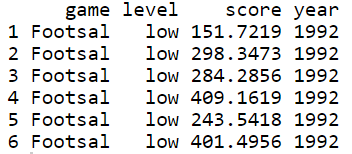## Plotting Grouped Boxplots

Here we are going to plot boxplots using geom_boxplot() methods.

Syntax of geom_boxplot(): geom_boxplot(mapping = NULL, data = NULL, outlier.shape = 19, na.rm = FALSE … )

Arguments:

• mapping: Set of aesthetic mappings
• data: data to be displayed in this layer
• outlier.shape: Displaying the outliers, NA – hides the outliers
• na.rm: dealing with missing values, TRUE – removes any missing values in data

### Plotting a Grouped Boxplot using the dataframe created

Using ggplot2 let’s plot a grouped box plot using the data frame created. Let’s visualize a boxplot between score and game, filled by level. Here we can compare each level with respect to games.

## R

 `# plotting a grouped boxplot``library``(ggplot2)`` ` `ggplot``(data, ``aes``(x = game, y = score, fill = level)) + ``geom_boxplot``()`

Output: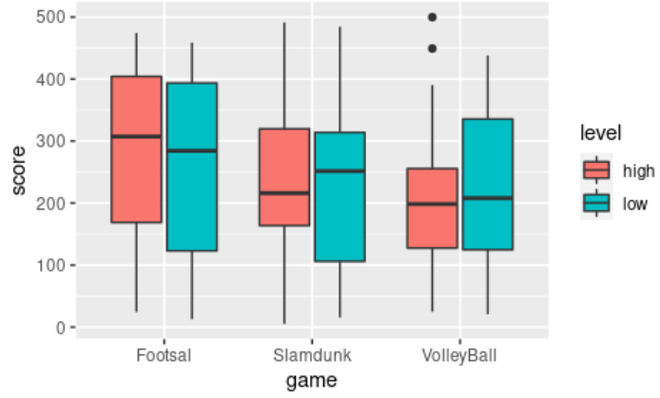### Plotting another boxplot using the dataframe created

Let’s visualize another boxplot between game and score, filled by level. This is the same as the above plot but with a changed axis which gives a clear comparison between each game with respect to the levels.

## R

 `# another grouped boxplot`` ` `ggplot``(data, ``aes``(x = level, y = score, fill = game)) + ``geom_boxplot``()`

Output: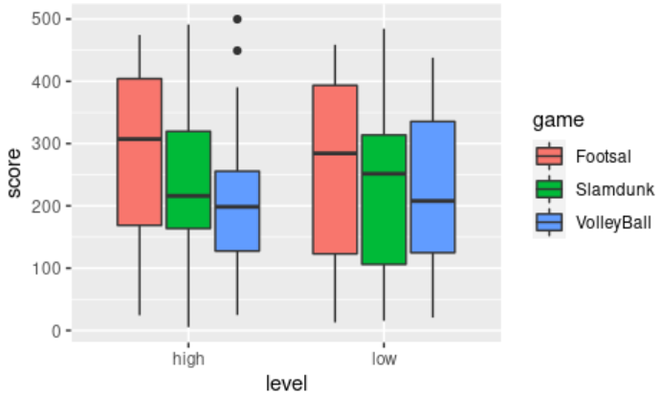## Adding Jittered Data Points to the Grouped Boxplot

Here we are adding jitter data points into the grouped boxplot using geom_point() method.

Syntax of geom_point(): geom_point(mapping = NULL, data = NULL,  position = “identity”, … )

Arguments:

• mapping – Set of aesthetic mappings
• data = dataset
• position – Position adjustment of points

### Adding jittered data points using geom_point( ) function and position_jitterdodge( ) argument

Let’s add jittered data points on the boxplot to visualize the spread of the data with respect to each game.

## R

 `# Adding jittered data points on the boxplot`` ` `ggplot``(data, ``aes``(x = game, y = score, fill = level)) + ``geom_boxplot``(outlier.shape = ``NA``)+``geom_point``(position = ``position_jitterdodge``(), alpha=0.3)`

Output: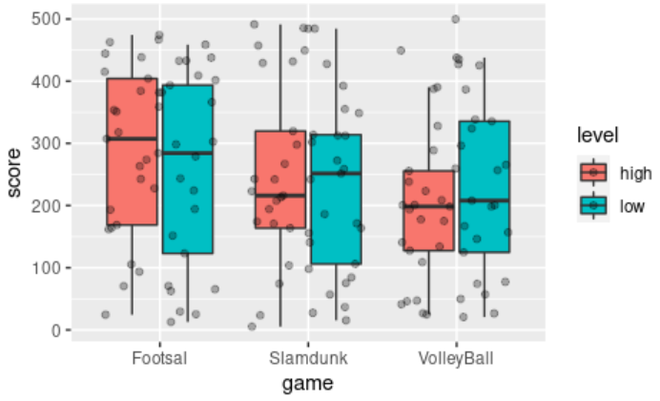### Plotting a Grouped Boxplot with jittered points with respect to performance in each game in each year

Let’s add jittered data points on the boxplot using geom_point( ) and facet_wrap( ) functions to compare the performance of scores in each game per year in different facets.

## R

 `# jittered points on boxplots in 3 facets by year`` ` `ggplot``(data, ``aes``(x = level, y = score, fill = game)) + ``geom_boxplot``()+``geom_jitter``(width = 0.1,alpha = 0.3) +``facet_wrap``(~year)`

Output: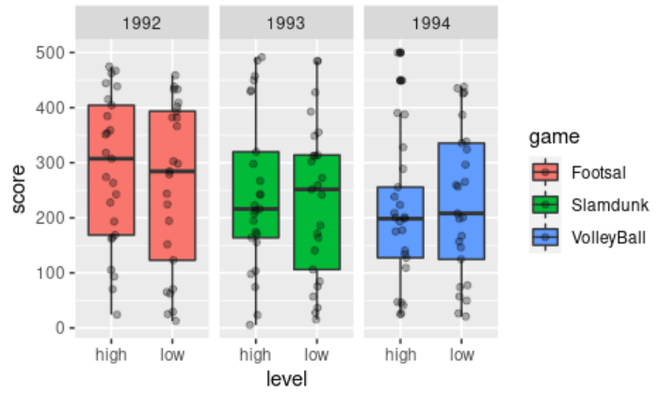My Personal Notes arrow_drop_up